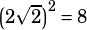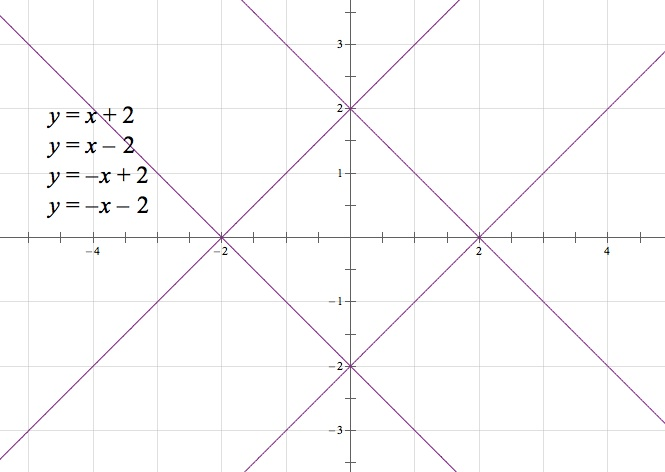Subject Test Question:

The area bound by the relationship |x|+|y|=2 is

A) 8
B) 1
C) 2
D) 4
E) there is no finite area.

How do you find this algebraically?

You need to capture all the combinations of negative and positive x and y. There are four equations to consider:

xy = 2
–xy = 2
x + (–y) = 2
x + (–y) = 2

Each of those is a line that can be represented in y = mx + b form. In the same order:

y = –x + 2
yx + 2
y = –x – 2
y = x – 2

Those make a square with vertices at (2, 0), (0, 2), (–2, 0), and (0, –2). The diagonals of the square are 4 long, making the sides of the squarelong. The area of a square with those side lengths is.